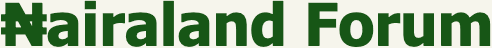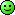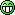#Welcome, Guest: Join Nairaland / LOGIN! / Trending / Recent / New
Stats: 2,779,138 members, 6,623,147 topics. Date: Thursday, 09 December 2021 at 08:21 AM

## Computer Science/programming Challenging Questions - Programming (3) - Nairaland

(1) (2) (3) (Reply) (Go Down)

 Re: Computer Science/programming Challenging Questions by airsaylongcon: 8:33am On Mar 02, 2016 ultimatex:All you have to do is register and start living life easy and smooth.Can you calculate the worst case scenario in big O notation of mergesort? Re: Computer Science/programming Challenging Questions by Fulaman198(m): 9:32am On Mar 02, 2016 I have a good problem for you guys to solve. I remember one of my professors years back giving me this one. Implement a method double getRoot(double x, int n, double tolerance)that returns the nth root of x to the given tolerance (plus or minus). Use a binary search algorithmAssume all parameters are positive. Avoid infinite loops when tolerance is too small.Make sure the code works when 0< x < 1. It should work for values of n in the hundreds, provided tolerance isn't too small.Only basic operations may be used (+, -, *, / etc.).Upload your function/method with a driver to prove that it works2 Likes Re: Computer Science/programming Challenging Questions by airsaylongcon: 9:40am On Mar 02, 2016 Fulaman198:I have a good problem for you guys to solve. I remember one of my professors years back giving me this one. Implement a method double getRoot(double x, int n, double tolerance)that returns the nth root of x to the given tolerance (plus or minus). Use a binary search algorithmAssume all parameters are positive. Avoid infinite loops when tolerance is too small.Make sure the code works when 0< x < 1. It should work for values of n in the hundreds, provided tolerance isn't too small.Only basic operations may be used (+, -, *, / etc.).Upload your function/method with a driver to prove that it worksExplain the function of the tolerance variable and how it works Re: Computer Science/programming Challenging Questions by DonSegmond(m): 2:01pm On Mar 02, 2016 Tosinosu2011:please i need a program that allow users to enter d number of tyres and it would be evenly divided through bicycle, car, and trycycle tyres. eg 9 tires will be: 1car, 1trycycle and 1bicycle. the program sud be in java. tenks I know this is for your homework, but I want to open your eyes that school might not be enough.I want you to know there is more out there, and that you can learn to do more and you should seize control of your life and constantly keep learning.Here is the solution in prolog.`:- use_module(library(clpfd)).solve(Tires, Cars, Tricycle, Bicycle):- Vars = [Cars,Tricycle,Bicycle], Vars ins 0..sup, Cars*4 + Tricycle*3 + Bicycle*2 #= Tires, label(Vars).`When we solve, we get the truth. With 9 tires, there are 3 solutions.?- solve(9, Car, Tricycle, Bicycle).Car = 0, Tricycle = 1, Bicycle = 3 ;Car = 0, Bicycle = 0, Tricycle = 3 ;Car = 1, Tricycle = 1, Bicycle = 1 ;Anyways, have fun with Java, but know there is more out there. 1 Like Re: Computer Science/programming Challenging Questions by Fulaman198(m): 7:44pm On Mar 02, 2016 Tosinosu2011:please i need a program that allow users to enter d number of tyres and it would be evenly divided through bicycle, car, and trycycle tyres. eg 9 tires will be: 1car, 1trycycle and 1bicycle. the program sud be in java. tenks I would write it for you, but you need to be more serious and read your textbook. Who is the author of your text? Re: Computer Science/programming Challenging Questions by Fulaman198(m): 11:28pm On Mar 02, 2016 Tosinosu2011:please i need a program that allow users to enter d number of tyres and it would be evenly divided through bicycle, car, and trycycle tyres. eg 9 tires will be: 1car, 1trycycle and 1bicycle. the program sud be in java. tenks If you attempt to write the program, I will help you with the rest. Re: Computer Science/programming Challenging Questions by airsaylongcon: 8:57am On Mar 03, 2016 I will post questions over the weekend as I am out of town guys Re: Computer Science/programming Challenging Questions by airsaylongcon: 2:50pm On Mar 07, 2016 Here we go guysConsider the following functionint t (){int q, output;output = 0;for ( q = 0; q < 5; q++ ){if ( ( q % 3 ) == 1 )output = output + q;elseoutput = output + 1;}return output;}What value is returned as a result of the call t () ? Re: Computer Science/programming Challenging Questions by codemarshal08(m): 5:44pm On Mar 07, 2016 airsaylongcon:Here we go guysConsider the following functionint t (){int q, output;output = 0;for ( q = 0; q < 5; k++ ){if ( ( q % 3 ) == 1 )output = output + q;elseoutput = output + 1;}return output;}What value is returned as a result of the call t () ?A call to this function will cause an infinite loop. it will never return as the value of q is never incremented Re: Computer Science/programming Challenging Questions by airsaylongcon: 5:54pm On Mar 07, 2016 codemarshal08:A call to this function will cause an infinite loop. it will never return as the value of q is never incrementedSorry that's a typo. My brain meant to type q++ while my hand typed k++. My apologies Re: Computer Science/programming Challenging Questions by codemarshal08(m): 6:02pm On Mar 07, 2016 airsaylongcon:Sorry that's a typo. My brain meant to type q++ while my hand typed k++. My apologiesOK. the return value is 8----------`1st comparison, q = 0 ----- 0%3 =====> 0, output = 0 + 1 = 1-----------12nd q = 1------1%3=====> 0. output = 1 + 1 = 2------------23rd q = 2------2%3=====> 0, output = 2 + 1----------------34th q = 3------3%3=====> 0, output = 3 + 1---------------45th q = 4------4%3=====> 1, output = 4 + q = 4 + 4-------8` Re: Computer Science/programming Challenging Questions by airsaylongcon: 6:11pm On Mar 07, 2016 codemarshal08:Ok the return value is 8That was straight forward. Well done Re: Computer Science/programming Challenging Questions by airsaylongcon: 6:15pm On Mar 07, 2016 Consider the following pseudocode.program main ()begininteger p, q, r, sp = 6q = 7r = 8s = fxn (p, q, r)print s, p, q, rendinteger fxn (integer a, integer b, integer c)beginif (a > 6) then b = 25c = a + breturn b + cendIf fxn uses call-by-reference, what values would be printed as a result of executing the pseudocode? Re: Computer Science/programming Challenging Questions by codemarshal08(m): 6:32pm On Mar 07, 2016 airsaylongcon:Consider the following pseudocode.program main ()begininteger p, q, r, sp = 6q = 7r = 8s = fxn (p, q, r)print s, p, q, rendinteger fxn (integer a, integer b, integer c)beginif (a > 6) thenb = 25c = a + breturn b + cendIf fxn uses call-by-reference, what values would be printed as a result of executing the pseudocode?Please, Does the if in the function have an [b]else [/b]part. Just want to be clear. I am not too convenient with pascal Syntax(i guess) Re: Computer Science/programming Challenging Questions by airsaylongcon: 6:36pm On Mar 07, 2016 codemarshal08:Please, Does the if in the function have an else part. Just want to be clear. I am not too convenient with pascal Syntax(i guess)I have edited the problem. I tried to put a space indicating that the if applied only to b=25 but some how that space vanishedRe: Computer Science/programming Challenging Questions by codemarshal08(m): 6:43pm On Mar 07, 2016 airsaylongcon:Consider the following pseudocode.program main ()begininteger p, q, r, sp = 6q = 7r = 8s = fxn (p, q, r)print s, p, q, rendinteger fxn (integer a, integer b, integer c)beginif (a > 6) then b = 25c = a + breturn b + cendIf fxn uses call-by-reference, what values would be printed as a result of executing the pseudocode?20, 6 , 7, 13 1 Like Re: Computer Science/programming Challenging Questions by asalimpo(m): 6:45pm On Mar 12, 2016 sad that this this thread dead. I wanted to learn from it. Re: Computer Science/programming Challenging Questions by Fulaman198(m): 8:05am On Mar 13, 2016 Tosinosu2011:please i need a program that allow users to enter d number of tyres and it would be evenly divided through bicycle, car, and trycycle tyres. eg 9 tires will be: 1car, 1trycycle and 1bicycle. the program sud be in java. tenks Did you ever figure it out? Let me know so I can help you with it. Re: Computer Science/programming Challenging Questions by Argonn(m): 11:57am On Apr 07, 2017 Please I need a pro to check this code for m. Its a program should accept input for user and count the number of vowels and consonants.The Code:v_count = 0c_count = 0alphabets = []vowels = ['a', 'e', 'i', 'o', 'u']consonants []for i in vowels:....vowels.append(chr(ord(i)-32))....#update the vowels list with capsfor i in range(65, 91):....alphabet.append(chr(i))....alphabet.append(chr(i+32))....#update the alphabets listfor i in alphabets:....if i not in vowels:........consonants.append(i)........#update the consonants listtext = input("Enter text:\t" )for letter in text:....if letter in vowels:........v_count += 1....elif letter in consonants:........c_count += 1....else:........continueprint("vowels: " + str(v_count))print("consonants: " + str(c_count)) Re: Computer Science/programming Challenging Questions by Kidstell: 1:55am On May 12, 2017 airsaylongcon:OK warm up with this. Remember that these are exam questions meant to be answered in approximately 2 minutes. Without the use of a compiler or stuff like thatConsider the following functiondouble p (double b, unsigned int e){if (e==0)return 1.0;elseif (even(e))return p(b*b, e/2);elsereturn p(b*b, e/2)*b;}How many multiplication are executed as a result of the call p(5.0, 12). Divisions SHOULD NOT be included in this totalAnswer = 8Given that I had to do it without paper. I think it's a very good brain task.Though I am yet to see another answer claiming that I am right I must also say that I noticed the condition for an even number input is never executed Re: Computer Science/programming Challenging Questions by Kidstell: 2:08am On May 12, 2017 airsaylongcon:Here we go guysConsider the following functionint t (){int q, output;output = 0;for ( q = 0; q < 5; q++ ){if ( ( q % 3 ) == 1 )output = output + q;elseoutput = output + 1;}return output;}What value is returned as a result of the call t () ?answer = 8 Re: Computer Science/programming Challenging Questions by Craigston: 11:48am On May 14, 2017 airsaylongcon:This one is deep. Old school Pascal programming. var a, b, c :integer;read (c) ;a:=1;b:=1;while a <10 dobeginb=b*a;a=a+1;If a = c then exitendFor the fragment of code above, which of the following statements about variables a and b must be true after execution of the fragment? A) (b=(c-1)!) AND (a>=c) B) (b=9!) AND (a=10)C) (b=10!) AND (a=10)D) ((b=10!) AND (a=10)) OR ((b=(c-1)!) AND (a=c)) E) ((b=9!) AND (a>=10)) OR ((b=(c-1)!) AND (a=c)) The answer is E.If the loop runs until the first a < 10, we cannot compute a b *= 10, but we must have done that from a = {1, 2, ..., 9}. At that point, a >= 10 is true after the last iteration.Similarly, we introduced a second termination condition that brings the iteration count closer, at a = c. This condition will be reached only if the integer read into c is less than 10. The same procedure above applies, with the range of a being`{a: 1 <= a < c}`during the execution of the loop's body. Then b = (c -1)! Is true after the loop exits, since a = c terminates the loop, just after a become equal to c. Re: Computer Science/programming Challenging Questions by Craigston: 12:00pm On May 14, 2017 Damn! I thought it was an active topic.